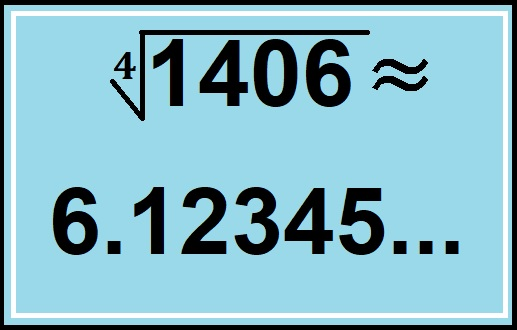# 1406 Has a Very Cool 4th Root

To find the 4th root of 1406, all you need to do is take its square root twice. The square root of 1406 is 37.4966665185. . .

Take the square root of that and you get a decimal starting with 6.12345…Here’s a little more about the number 1406:

• 1406 is a composite number.
• Prime factorization: 1406 = 2 × 19 × 37.
• 1406 has no exponents greater than 1 in its prime factorization, so √1406 cannot be simplified.
• The exponents in the prime factorization are 1, 1, and 1. Adding one to each exponent and multiplying we get (1 + 1)(1 + 1)(1 + 1) = 2 × 2 × 2 = 8. Therefore 1406 has exactly 8 factors.
• The factors of 1406 are outlined with their factor pair partners in the graphic below.1406 is the sum of the first 37 EVEN numbers because 37 × 38=1406.

1406 is the hypotenuse of a Pythagorean triple:
456-1330-1406 which is (12-35-37) times 38

This site uses Akismet to reduce spam. Learn how your comment data is processed.